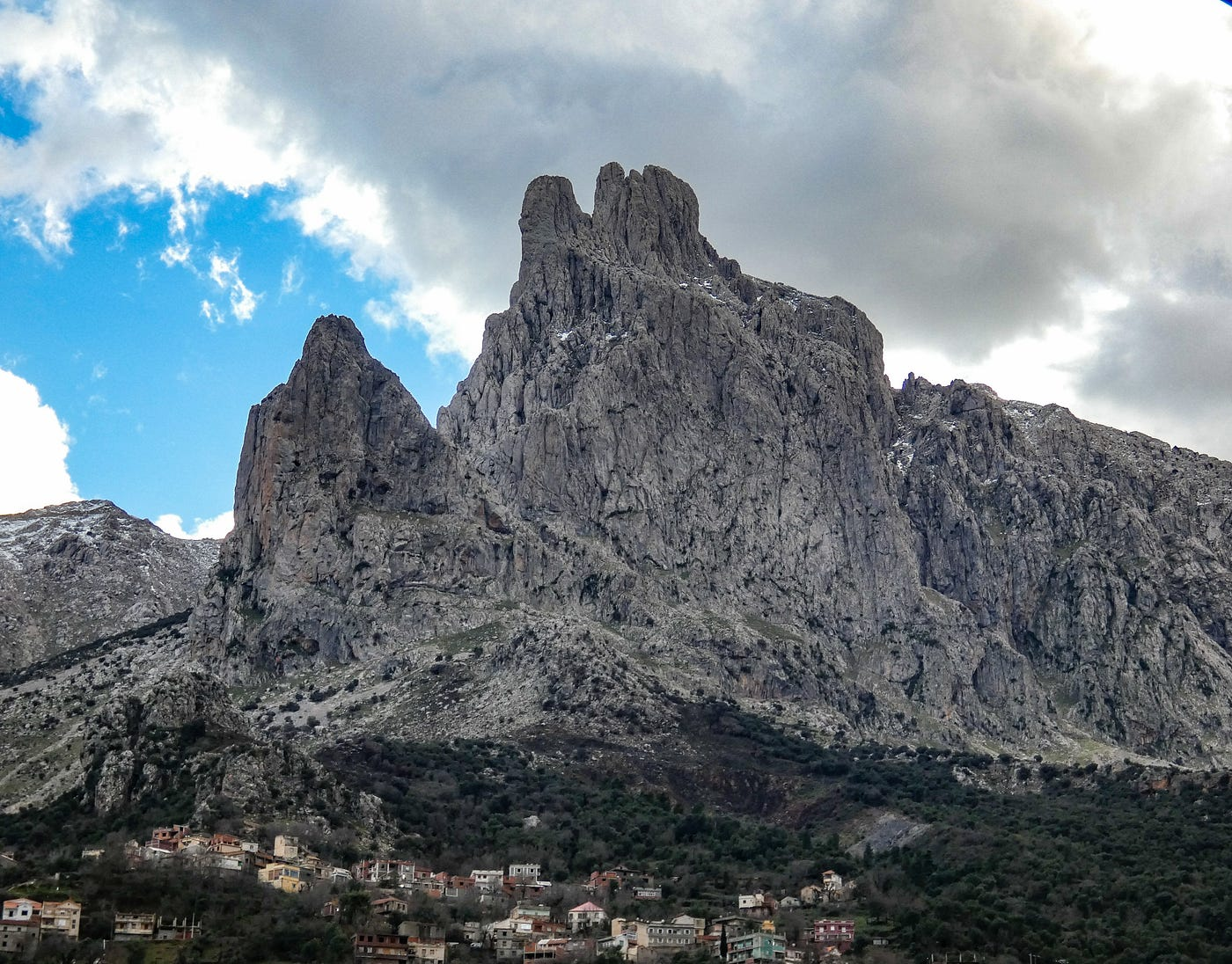# Double Deep Q-NetworksMain du juif mountain in Algeria. Taken by me

## Bellman Optimality Equation

The goal of the agent is to find a policy that satisfies the Bellman optimality equation for each state s and action a.

## What are the issues?

Both the Q-values and the Q-targets are computed using the same DNN. The DNN’s goal is to reduce the lack between each Q-value and its Q-target by updating its parameters. And here is all the problem. When we update the DNN parameters, we make the Q-value closer to the Q-target but the Q-target is changed and moves in the same direction than the Q-value since we use the same DNN to compute both the Q-value and the Q-target

## Double Deep Q-Networks algorithm

To overcome this problem, we use the Double Deep Q-Networks algorithm. The idea is quite simple: Instead of using one DNN to compute both the Q-values and the Q-targets, we use two DNNs. The first one computes the Q-values and the second one the Q-targets. After a certain number of experiences, we update the parameters of the Q-target neural network by copying those of the Q-value neural network. We understand that these two DNNs have the same architecture.

## Conclusion

The DDQN allows the agent to converge quickly and to be more accurate. In this post, we only explained the DDQN algorithm. I will try to prepare another post where I will compare the performances of a standard DQN agent against a DDQN one. I hope you enjoyed this post. Please let me know if you have any questions or comments.

--

--## Amrani Amine

5th year computer science and statistics engineering student at Polytech Lille, I am fascinated by machine learning and Graph Neural Networks.